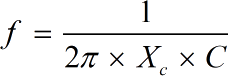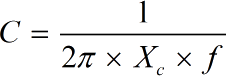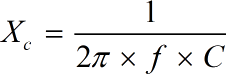Request a Tool

# Capacitive Reactance Calculator

The capacitive reactance tool can be used to calculate the resistance of a capacitor in an electric circuit.

Frequency
0

#### Formula• f = Frequency
• C = Capacitance
• Xc = Capacitive Reactance

#### Defination / Uses

A sinusoidal wave's frequency is defined as the number of complete oscillations made by any wave constituent per unit of time. Its SI unit is Hertz(Hz).

Capacitance
0

#### Formula• C = Capacitance
• Xc = Capacitive Reactance
• f = Frequency

#### Defination / Uses

The ability of a capacitor to store energy in an electric field is referred to as capacitance. An electronic component known as a capacitor is used to store this energy. The symbol 'C' is used to represent capacitance. The capacitance C and the voltage differential V applied across the capacitor determine the amount charged. When a constant voltage is applied to the capacitor, it creates a pair of plates.

#### Output

Capacitive Reactance
0

#### Formula• Xc = Capacitive Reactance
• f = Frequency
• C = Capacitance

#### Defination / Uses

The capacitor applies a short circuit signal path to any unnecessary frequency signals on the output terminals, it is perfect for use in AC filter circuits or DC power supply smoothing circuits to lessen the impact of any unwanted ripple voltage.

This is a useful tool for estimating a capacitor's so-called resistance in an electric circuit. Estimating the capacitive reactance of any capacitor is not difficult at all.

#### How this tool is helpfull

Because the capacitor applies a short circuit signal path to any undesirable frequency signals on the output terminals, it is excellent for use in AC filter circuits or DC power supply smoothing circuits to lessen the impact of any unwanted Ripple Voltage. Estimating the capacitive reactance of any capacitor is not difficult at all. You just have to fill the required fields like capacitance and frequency to get accurate answer OR you can use the given formula. As compared to manually calculation this calculator will save your time.